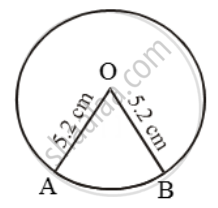Share

# The perimeter of a sector of a circle of radius 5.2 cm is 16.4 cm. Find the area of the sector. - CBSE Class 10 - Mathematics

ConceptProblems Based on Areas and Perimeter Or Circumference of Circle, Sector and Segment of a Circle

#### Question

The perimeter of a sector of a circle of radius 5.2 cm is 16.4 cm. Find the area of the sector.

#### Solution

Let OAB be the given sector. Then,

Perimeter of sector OAB = 16.4 cm⇒ OA + OB + arc AB = 16.4 cm

⇒ 5.2 + 5.2 + arc AB = 16.4

⇒ arc AB = 6 cm

⇒ / = 6 cm

∴ Area of sector OAB = \frac { 1 }{ 2 }

= \frac { 1 }{ 2 } × 6 × 5.2 cm^2 = 15.6 cm^2

Is there an error in this question or solution?

#### Video TutorialsVIEW ALL 

Solution The perimeter of a sector of a circle of radius 5.2 cm is 16.4 cm. Find the area of the sector. Concept: Problems Based on Areas and Perimeter Or Circumference of Circle, Sector and Segment of a Circle.
S